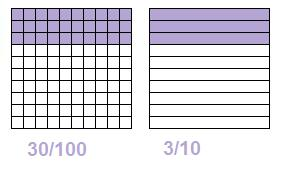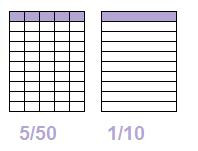### Writing fractions in their simplest form

Divide numerator (top) and denominator (bottom) by a number that is divisible by both (find a factor of both)

#### Example 1.     4/10 = 2/5

(dividing top and bottom by 2)#### Example 2.     5/20 = ¼

(dividing top and bottom by 5)#### Example 3.     30/100 = 3/10

(dividing top and bottom by 10)#### Example 4.     5/50 = 1/10

(dividing top and bottom by 5)to: